SELF 4 S.B. Karavashkin and O.N. KaravashkinaLet us go on analysing, how Fermi built the Schroedinger equation, and mark that, taking the integral of action under condition of its constancy, Fermi came to the following expression for the group velocity: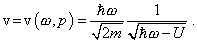(16)
 “Such monochromatic wave satisfies the equation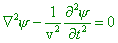(17)
 whose particular solution is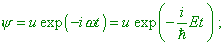(18)
 here the monochromatic meaning requires to take the constant frequency” [8, p. 21]. Let us stop and analyse some basic aspects of the yielded solution (18). As we see, the modelling equation (17) that Fermi wrote but not derived is the outward form of the standard wave equation of classical wave physics. The only difference is that in the denominator of the coefficient in the time derivative in (17), we have the group velocity. Well, in accordance with (16), Fermi himself determined this velocity dependent on the frequencies of the wave packet. And the solution of (17) is the monochromatic wave. But the wave packet does not vary harmonically, as (18) does. Thus, we see the wave equation to be introduced artificially, and incorrect transitions retaining only outward attributes that could agree this construction with Bohr’s solutions yielded on the basis of classical physics. And the farther derivation is developed the more this discrepancy is revealed. Having showed the quasi-wave equation and its quasi-wave solution, Fermi substitutes (18) into (17) and passes to the expression for unknown coefficients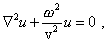(19)
 “or, noting (16),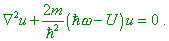(20)
 Substitutingu with the help of correspondence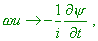(21)
 we come to the time-dependent Schroedinger equation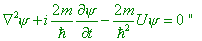(22)
 [8, p. 21]. And it appears that “in case of solution (18) we have the equation for stationary states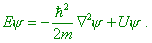(23)
 This equation has a sense only for the states with fixed energy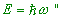[8, p. 21]. Thus, it appears that, proceeding from (18), by way of formal transformations, Fermi yields (22) and derives from it that (18) satisfies another equation, (23). But not only this we can see in this construction of the Schroedinger equation. Let us draw our attention that, accordingly to Fermi’s additional stipulation, the equation for stationary states (23) is true only for a particular relationship between the frequency and energy. At the same time, we can see it in no way in the transformation and in the very expression (23). In particular, should we consider a classical model with resonance properties, then immediately from the mathematical description we would yield the resonance conditions. But the basic wave equation (17) is the equation of most general kind, it admits continuous, not discrete spectrum of frequencies. The Schroedinger equation (22) derived immediately from (17) only reflects this fact. And we mean that we study just the wave packet, not monochromatic wave. What discrete frequencies can we discuss when Fermi states that the de Broglie wave describes a packet? Hence, the Schroedinger equation (22) and the equation for stationary states (23) do not describe the states with discrete level of the energy, and the introduced addition is artificial. And this is really so. Passing in the third lecture to the particular problems, for closed line Fermi adds a stipulation to the modelling equation: “The condition of periodicity requires, the function u to have the shape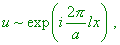(24)
 where l takes any integer values (positive, negative and zero)” [8, p. 27]. But this condition has been carried over just from classical wave physics. In quantum mechanics, when introduced the uncertainty principle by Heisenberg, we may not speak of any strong periodicity. And if the levels were already quantified, why have we to quantify them additionally? So, proceeding from the principle of quantification, we violate the solutions that have been constructed namely noting this principle, as with the periodicity violation, as well as if the determinacy disappears, if the pattern of wave function is statistic, we will loss the condition of discretisation for the energy levels that were introduced a priori into the statement of problem as the Planck postulate of energy quantification. “And it seems to me, it will not be a mistake to say, as de Broglie as Schroedinger sought first of all the mathematical tool, i.e. sought such construction for the mathematical side of theory in which the selection of discrete values would be inherent, doing not caring much, which physical meaning would they have to attribute to the values that will be involved into this mathematical tool, with exception of the very discrete values that, apparently, they had to interpret as E (energy) either(frequency) - this all was done for it. In seeking this tool, undoubtedly, the decisive part belonged to the circumstance that in classical physics such tool existed (! - Authors). These were so-called problems of eigenvalues, i.e. in essence the wave equations with the boundary conditions. They solved the problems of natural (discrete) vibrations of strings etc. There, of course, the physical meaning of all mathematical symbols was clear. There, this tool appears in application of general principles of mechanics or electrodynamics to particular issues. For the boundary problems, there is typical to select the integer numbers that provide the existence of solution. What Schroedinger did, what he guessed (! - Authors) was significant in the following relation. In the string, the discrete numbers are selected not so much by the equation as by the boundary conditions. (Factually, this was a great mistake of mathematicians and mechanics of that time that they first generalised the equations of particular problems where discretisation was determined namely by equations, - doing not solving them in due way, - and after generalisation they substituted the phenomenology of mathematical modelling, moving away even farther from the exact solutions. We showed it in our papers on modelling of complex vibration systems.) And where can we take these boundary conditions for an atom, what have we to catch on? Well, Schroedinger has put his attention (mathematician knew it long ago) that there exist such differential equations which need for discretisation only natural conditions - such, for example, as integrability of the squared module of solution and its finiteness at the special points of equation. Just in this way Schroedinger came to his equation, and he himself said, he did not expect such staggering effect from his work” [10, p. 330].

Contents: / 1 / 2 / 3 / 4 / 5 / 6 / 7 / 8 / 9 / 10 / 11 / 12 / 13 / 14 / 15 /